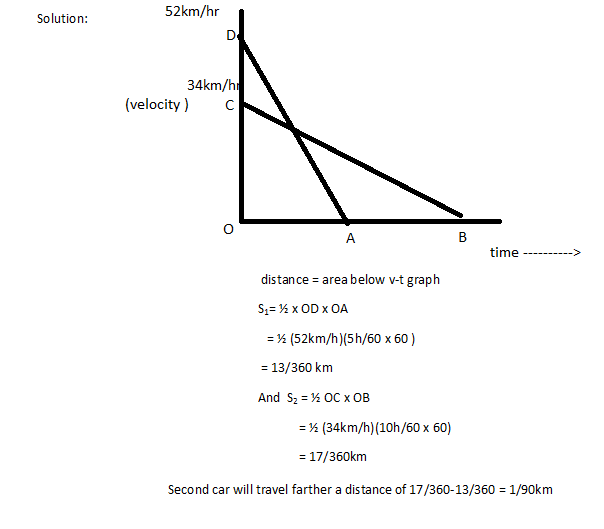Home >> Class 9 >>

# Motion Class 9 Science Chapter, Explanation, Notes, Question Answers

## CBSE Class 9 Science Chapter 8 Motion, Explanation, Examples, Question Answers

Motion CBSE Class 9 Science Chapter 8 - Complete explanation and Notes of the chapter 'Motion'. Topics covered in the lesson are Rest and Motion, Acceleration, Types of Motion, Distance Time Graphs, Scalar and Vector Quantities, Velocity Time Graphs, Distance and Displacement, Derive Three Equations of Motion, Uniform & Non Uniform Motion, Circular Motion, Speed, Velocity. Given here is the complete explanation of the chapter, along with all the important questions and NCERT solutions to book questions have also been provided for the ease of students.

### Class 9 Science Chapter 8 - Motion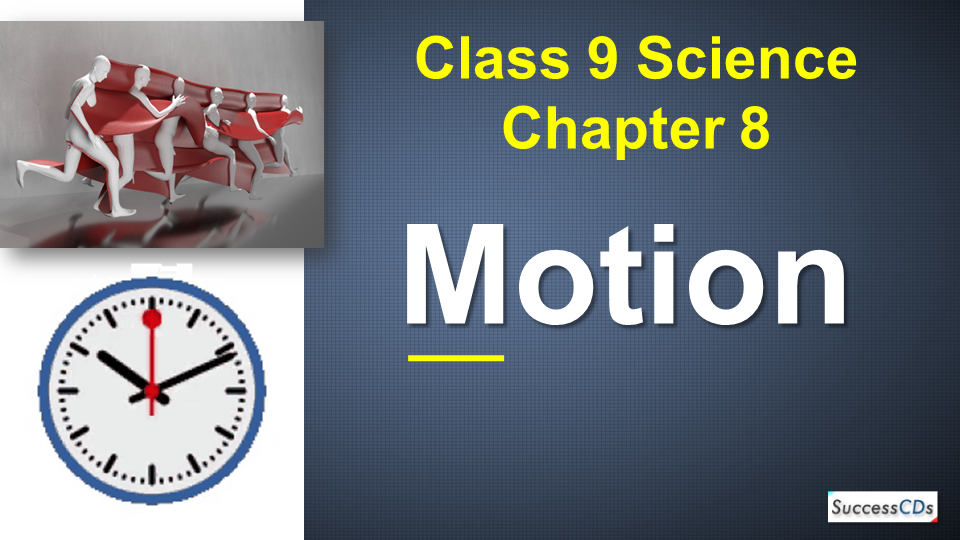## Motion Class 9 Science Chapter Introduction

You all use common things around you that are moving like if you see around you, observe air moving around you, like you have clocks with the hands moving, you all know that day and night is caused because of motion of Earth around the Sun, even seasons are caused because of it. So we are going to study in detail what exactly is the term motion.

To know more about motion or rest we should first get familiar with the term reference point or stationary object. To comment about the state of any object, we have to consider a reference point or stationary object or surroundings. This reference point, stationary object does not change its position.

For Example: If we consider a pole and a car, then a pole will be stationary as it can’t change its position but the car can change. So, in order to say that car is at rest or motion we need to consider its motion with respect to that of pole or any other stationary object. This stationary object can also be called as reference point.

## Rest and motion

For this let us take different examples to understand it:
Let us consider a car standing in front of house. Let’s say first it is at position A then after some it moves to position B. That means it has changed its position with respect to stationary object that is house.And if that car keeps on standing at position A that means it hasn’t changed its position with respect to house that means it is at rest so here we define the terms rest and motion.

Rest

When a body doesn’t change its position with respect to its surroundings or reference point

Motion

When the body changes its position with respect to its surroundings or reference point.
So, we can say that when any object is moving or in motion it possess following characteristics as given below.

Characteristics of moving object

• The moving object changes its position with time

Now we have seen that motion of car can be easily seen that is we don’t have to concentrate much but to know the movement of arm of clock let’s say hour hand we need to keep check this is because some motion is that fast that we can see it happening, on the other hand some motion is so slow that it can’t be seen clearly.

We can elaborate it as: Our wrist watch has three hands: minute, seconds and hour hand .out of them second hand moves fast that its motion can be seen but in order to see the motion in hour hand and minute hand we have to keep the track as the motion in them is comparatively slow.

### Types of Motion

We come across different types of motions like

• Linear
• Rotational
• Circular
• Vibratory

Let us explain them with examples:
Linear

• We all when drive on road mostly the roads are straight so, that motion where we move in a straight line is called linear motion

Rotational

• You all know the earth rotates on its axis which causes day and night that movement is rotational motion because it is rotating on its axis so, we can define as the rotational motion when the body rotates about a fixed axis.

Circular

• You all encounter round bouts on road and when you are driving you can’t drive straight through it, you have to take the curved path that is the circular motion. So, if body travels along a curved path

Vibratory

• You all must have played with the instrument guitar. So, what happens when you strike it with your finger its string starts vibrating and thus the sound is produced. That motion is vibratory as it is caused due to vibratory motion of particles so it is when body show to and from movements.

### Scalar and vector physical quantities

We study so many physical quantities like distance, velocity and many more. All these quantities are broadly classified under two categories that is scalar or vector depending upon where they give the complete information about the magnitude (value) and direction or give the incomplete information like only direction or only value.

Scalar Quantities

The quantities that depend upon magnitude and not the direction is called scalar quantities. They are represented as their own symbol.
For Example: If you travel to Delhi to your relative’s place and if someone asks you about the distance what do you reply. We say it was like 250km run from Chandigarh. You don’t tell him that 25km towards east, then west. We just say 250km that means we describe it only in magnitude and we don’t specify directions. So, it is a scalar quantity.

Vector Quantities

The physical quantities that depends upon magnitude as well as direction. They are represented by putting arrow on their symbol.
For Example: Now if you travel a straight short path in a specific direction then we can say that I travelled 25km towards east. So, in this case direction is specified so it falls in the category of vector quantity.

### Distance & Displacement

Let’s take an example to understand Distance

• Consider a boy going to school, then from school going to a market, then going to a friend’s place to return his book and then coming back home let’s say he travels 2km from home to school then 1 km to market then 1 km to friends place then 2 km to reach home. Then the total path travelled by boy is 2+1+1+2=6 km. So, the sum total of path he travels in any direction gives us the distance.

Distance

It can be defined as total path covered by body in any direction. It is a scalar quantity.

• Formula: distance = total path travelled
• Unit - meter/second
• Bigger unit: Km/hour
• 1 km = 1000m

Characteristics of distance

• It can never be zero: it is not possible that body moves but the distance is zero
• If a body travels different paths then total distance is calculated by simply adding the magnitude of all the paths
• It is a scalar quantity

Let us understand displacement now

Let’s say there were 3 friends Ram, Shyam and Geeta. Now ram has to go to Geeta’s place but he doesn’t know the house so what he plans He first went to Shyam place and then with shyam he went to Geeta’s home. In doing so he has to travel long distance first to Shyam house and then to Geeta. But if he knew the house of Geeta then he would have taken a short route and in specific direction that short route in particular direction is called displacement.

Displacement

• It is the shortest route travelled by body in given direction
• It is represented as S
• It is a vector quantity
• It can be zero: whenever a body starts from one point and returns to the same point the displacement is zero.
• If body travels different paths then total displacement is calculated by adding different vectors

Let us do a problem on distance and displacement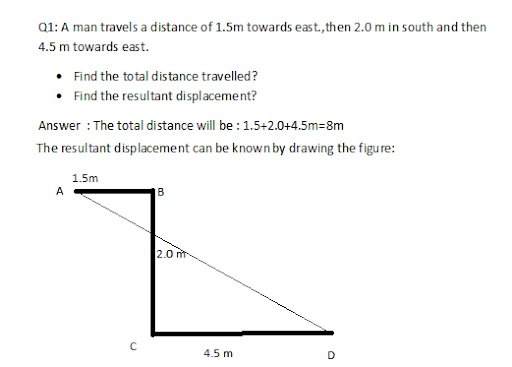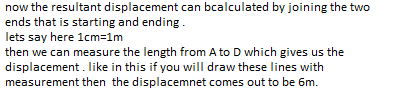### Uniform and non uniform motion

Uniform motion

When body covers equal distances in equal intervals of time, the body is said to home uniform motion.

Example: A train running at a speed of 90 km/hr.

Let’s say a train is travelling to Delhi and it covers the same distance in the same intervals of time like:

 Distance 90 km 180km 270km 450km Time 1 hr 2hr 3hr 4 hr

So here we see that train travels equal distance of 90km in each hour .so it is said to be moving with uniform motion.
The graph involved in it is given as: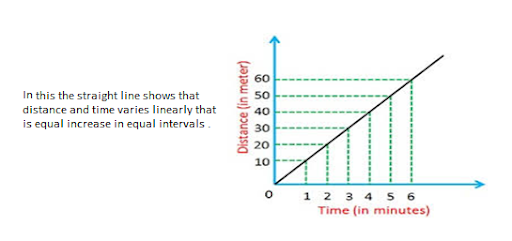Non-uniform motion

When body covers unequal distance is equal intervals of time.

Example: when brakes are applied to speeding car.

Let’s say A car travelling to Delhi covers 40km in one hour and then due to huge traffic on road travels 25km in another hour and then in another hour takes a break for having lunch, then speed up the car and travels 50km in another hour. It can be shown as:

 Distance 40km 65km 115km Time 1hr 2hr 3hr

So, in this unequal distance is travelled in equal intervals of time.
The graph drawn to show non uniform motion is: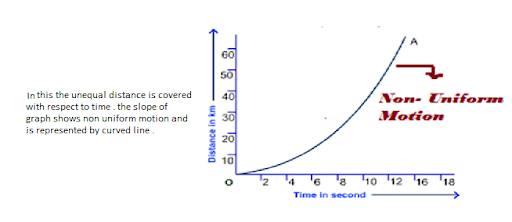### Speed

We all often say to a driver that drive carefully or drive slowly. If he drives slowly we reach the destination late and if he drives fast we reach the destination early. This is all due to speed which actually means how much distance we are covering in given time.

It is defined travelled by body with respect to time in any direction.

• It is Represented as V
• Formula 🡺 speed = Distance/time
• speed= distance /time
• Unit => m/sec or m/sec or m sec-1 or km/hr)
• It is a scalar quantity

Speed of any object can also be measured at any instant of time with the help of speedometer, whereas. The distance travelled by car is measured by Odometer.

Speed is of Three Types:

• Uniform speed
• Non uniform speed
• Average speed

Average Speed

In order to understand it let’s take an example: If we travel to Delhi in 4hours and if your friend asks you about your speed you reply 60km/hr or 40km/hr. This is average speed. As you don’t reply that in first hour it was 50 then in another hour it was 45km/hr. We actually calculate it by measure of total distance of your destination from where you started by total time that you took to reach.

It is defined as the total distance travelled by body divided by total time taken to cover distance.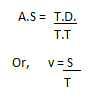Let’s do problems based on average speed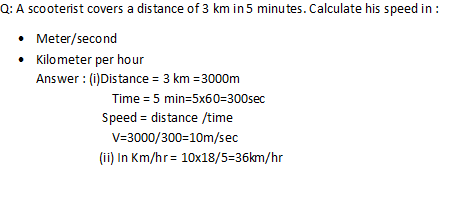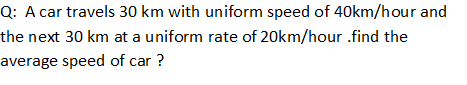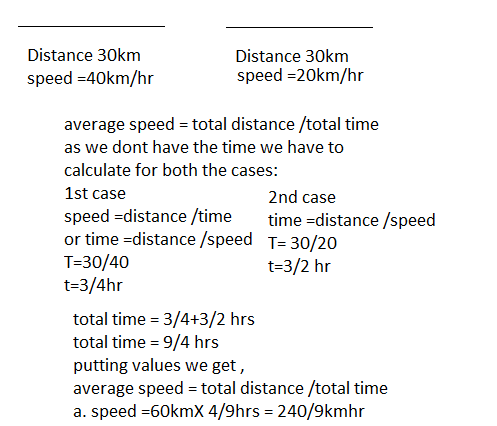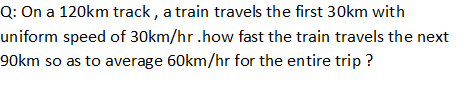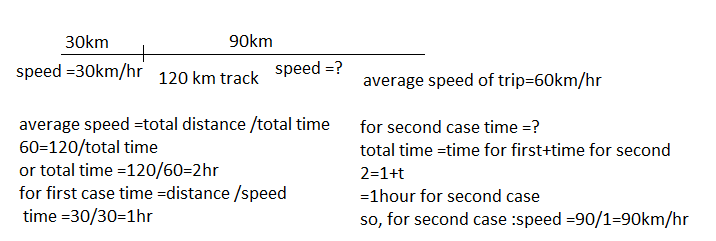### Velocity

The speed gives us the information about the distance with respect to time but doesn’t specify the direction so we are in immediate need of physical quantity that gives the information as speed and also specifies the direction so here comes velocity.
It is the distance covered by a body per unit time in a given direction. Or “Speed in given direction”

• Units🡪 m/s or km/hr (meter)
• It is a vector quantity
• It is a complete quantity

Types of velocity

• Uniform velocity
• Non uniform velocity
• Average velocity

Uniform Velocity
Let’s take an example suppose we are travelling to Delhi and we are covering the same distance like in each hour we cover 60 km in specified direction then the body is said to be travelling with uniform velocity.
When body covers equal distances is equal intervals of time in a straight line (given direction).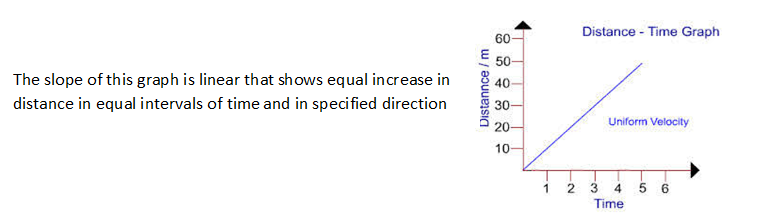Non-uniform Velocity
If we are travelling to Delhi and due to mending of roads or any other reason there is huge traffic due to which we are not able to take the straight line direction and also the speed doesn’t remain same then the velocity is said to be non uniform velocity.
When body covers unequal distances in equal intervals of time not in a straight line (not in given direction).
Example: A car running towards north on busy road with variable velocity.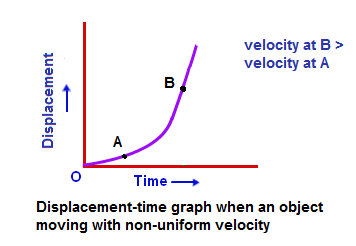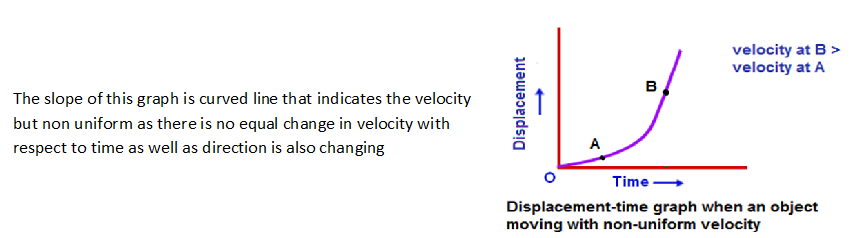Before studying average velocity let’s take in to account about the concept of initial and final velocities
Suppose a scooter is at rest and then we start it and now it is moving at a speed of 20m/sec. Then the velocity which it possesses earlier is initial velocity and what it possesses now is its final velocity. That is earlier at rest so zero and now moving at 20m/sec so final velocity is 20 m/sec.
Similarly, If I say that scooter is moving at 20m/sec and then its speed increases to 40m/sec. Then its initial velocity is 20m/sec and final is 40m/sec
In the same way, if I say scooter is moving with 40m/sec and then suddenly brakes are applied and it stops. Then in this case initial velocity is 40m/sec and final will be zero as it stops.

• Initial velocity is denoted by ‘u’
• Final velocity is denoted by ‘v’

Average velocity

It is actually an arithmetic mean of initial velocity & final Velocity for given time. Or it can be defined as total displacement per unit total time taken.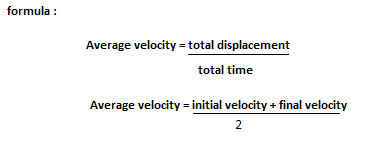### Acceleration

Whenever the velocity increases or decreases with time, then another quantity comes in to notice and that is acceleration.
Is defined as the rate of change of velocity with time.• Units = m/sec 2 or m sec -2
• It is a vector quantity

• If velocity increases with time => +ve Acceleration
• If velocity decreases with time => - Acceleration or retardation ode-acceleration

Types of acceleration

• Uniform acceleration
• Non uniform acceleration
• Retardation

Let’s explain them

Uniform Acceleration

Suppose we are travelling to Delhi from Chandigarh and our velocity increases in equal amounts like initially it was 50m/sec then it becomes 70m/sec, then 90m/sec in equal intervals of time and in a straight line then the acceleration comes in to play as velocity is undergoing a change.

When velocity of a body undergoes equal changes in velocity in equal intervals of time in straight line.

Example: Freely falling body

Non-uniform Acceleration

Suppose in a similar as we took for uniform acceleration if increase or decrease in velocity is not regular and not in the specified direction, then it falls in the category of non uniform acceleration.

When velocity undergoes unequal changes in equal intervals of time and the direction may not be a straight line

Example: Body moving with variable velocity

Retardation

Acceleration is said to be retardation when velocity decreases with time.

Example: Slowing down of car

Newton’s equation of motion

Newton gave three equations of motion that is followed universally. These equations are: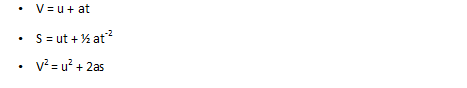In these equations V= final velocity, U=initial velocity,

S = distance or displacement, a= acceleration and t=time.

### Distance time graph

Like we took so many examples above so, in any example if we plot distance versus time we get the important information from it.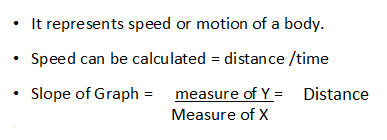When d-t graph is parallel to time axis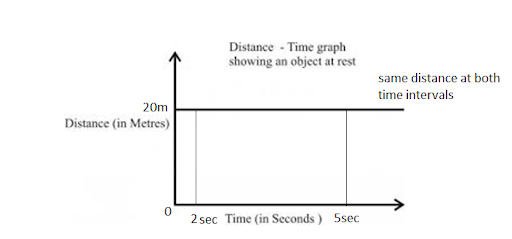In this body is not changing its position with respect to time that shows body is stationary. Like if we take the markings on the axis, we see that in any time the distance covered is exactly the same.

So, the information that we get, is:

• The body is stationary

When d-t graph is straight line not parallel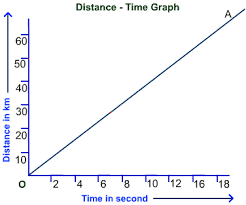This shows the distance covered is directly proportional to time that shows body is moving with [uniform speed]
If we look at the markings carefully we observe, that when time is 2 sec then distance is 10m, when time is 4 sec the distance is 20m. We can say there is an equal increase with equal intervals of time.
So, the information we get is:

• Body is moving with uniform speed

When d-t graph is not a straight line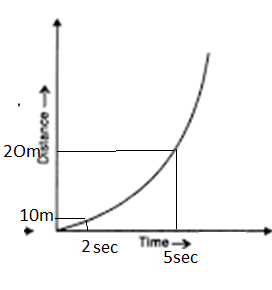If we look at the markings carefully we observe, that when time is 2 sec then distance is 10m, when time is 5 sec the distance is 20m. We can say there is an unequal increase with equal intervals of time.
So, the information we get:

• This shows body is moving with non uniform speed.

### Velocity Time -Graph

Like we took so many examples above so, in any example if we plot velocity versus time, then we get the important information from it.

• It represents acceleration
• The area enclosed under graph gives displacement

When v-t graph is || to time axis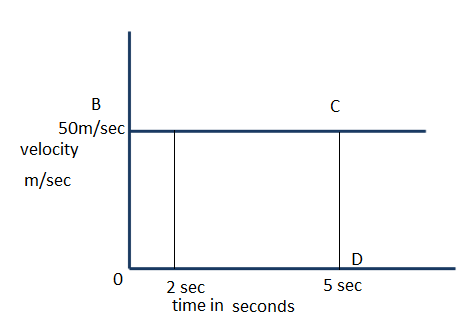In this body is not changing its velocity with respect to time that shows body is moving with the same velocity. Like if we take the markings on the axis, we see that in any time the velocity is exactly the same.
So, the information that we get, is:

• The body is moving with constant velocity
• Acceleration = change in velocity/time

=0/5-2=0m/sec2

• The displacement =area under v-t graph

S= area of rectangle
= OBCD
= L x B= 50 x 5 = 250m

• This shows body is starting from rest & then its velocity increases at uniform rate.

When v-t is straight line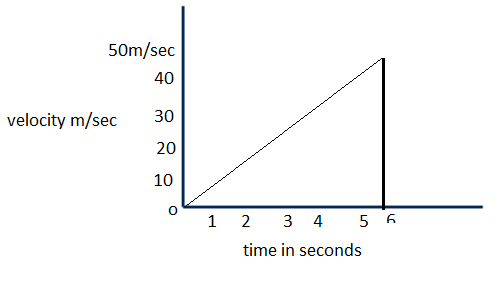This shows velocity is directly proportional to time that shows body is moving with [uniform velocity]

If we look at the markings carefully we observe, that when time is 1 sec then velocity is 10m/sec, when time is 2 sec the velocity is 20m/sec. We can say there is an equal increase with equal intervals of time.

So, the information we get is:

• Body is moving with uniform velocity
• Acc =equal to the slope

a=v-u/t
a=50-0/6=50/6=8.3m/sec2

• Displacement = area of triangle

S=1/2 Lx B
S=1/2 x 6 x 50=150 m

When v-t is straight line but body does start from rest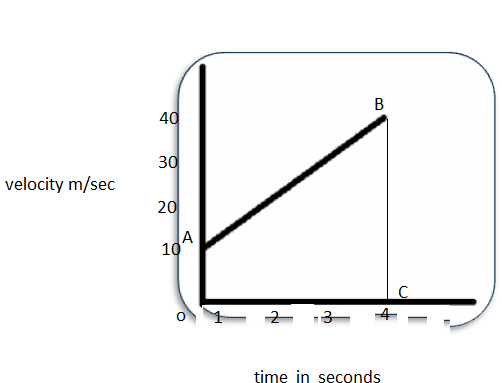This shows velocity is directly proportional to time that shows body is moving with [uniform velocity] but here in this body is not starting from rest, it has already acquired a velocity of 10m/sec.
If we look at the markings carefully we observe, that when time is 1 sec then velocity is 10m/sec, when time is 2 sec the velocity is 20m/sec. We can say there is an equal increase with equal intervals of time.
So, the information we get is:

• Body is moving with uniform velocity but not starting from rest .
• Acc =equal to the slope

a= v-u/t
a=40-10/4=30/4=7.4m/sec2

• Displacement = area of trapezium

S= ½ (OA+ BC) x CO
= ½ (10 +40) x 4
= ½ x 50 x 4 =100m

### Derive equations of Motion => [Graphically]

Ist equation:     V = u + at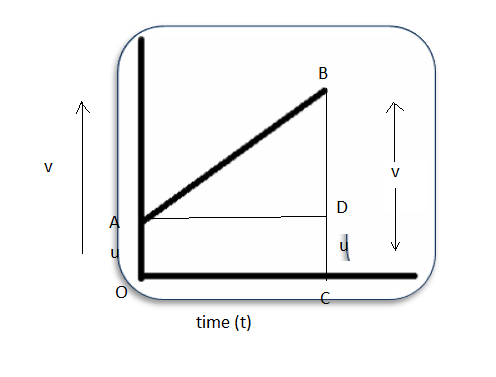S= area of trapezium
S= (sum of two parallel sides) x height
S= (u+v) x t

Substituting the value of ‘v’ from the first equation of motion, we get
S= (v+u)(v-u/2a)
S= (v2-u2)/2a
Or, v2-u2= 2as
Hence proved.

### Circular Motion

You must have encountered roundabout while travelling on a road or you must have seen the athletes running in a circular track there the motion is not in a straight line there one has to move in circles. This is circular motion.
The motion of a body around a circular path with uniform speed is called circular motion”.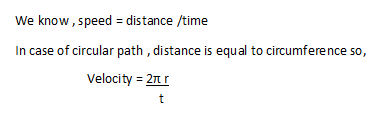Please note that: When body revolves in circular path it moves with uniform speed but its direction changes continuously . Due to which we can say it has non uniform velocity ,so this on uniform velocity give rise to acceleration therefore the motion is said to be accelerated motion .

1. A scooter acquires a velocity of 36 km/hour in 10 seconds just after the start. Calculate acceleration of scooter?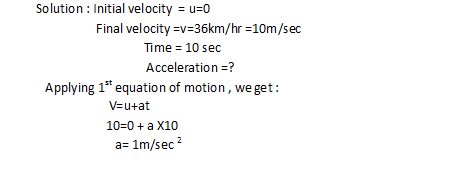1. A moving train is brought to rest within 20 seconds by applying the brakes. Find the initial velocity if retardation due to brakes is 2m/sec 2?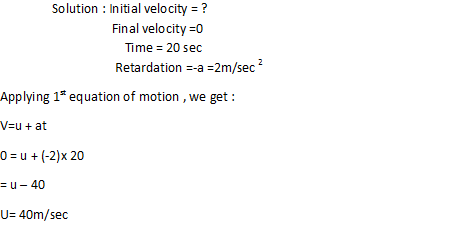1. A body starts to slide over a horizontal surface with initial velocity of 0.5 m/sec. Due to friction its velocity decreases at the rate of 0.05m/sec2. How much time will the body takes to stop?

Solution:1. A scooter moving with a speed of 10m/sec is stopped by applying brakes which produce uniform acceleration of -0.05m/sec. How much distance will it cover before it stops?

Solution: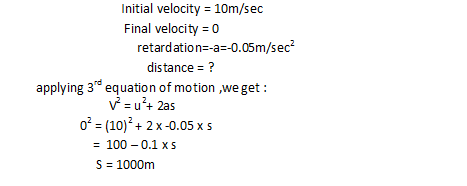### NCERT Book solutions

Q 1. Why blades of fan are said to have an acc. motion?
A. When the blades of fan rotate they rotate in circular path although they rotate with uniform speed but direction of blades continuously changes. Therefore, we can say that they are rotating with non-uniform velocity.
We also know, the rate of change of velocity is acceleration. So, we can say blade of fan possess acc. motion as there is continuous change velocity with time.

Q. Joseph jogs from one end A to another end B of a straight 300m road in 2 min 30 seconds and then turns around and jogs 100 m back to point C in another 1 minute. What are Joseph’s average speeds and average velocities from (a) A to B (b) A to C?
Solution: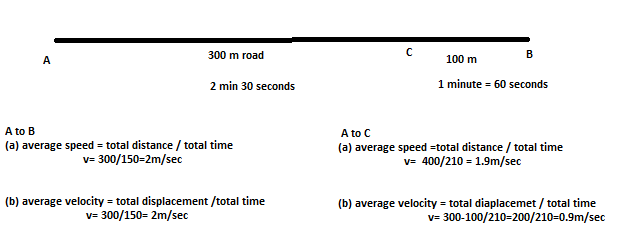Q. Abdul while driving to school computes the average for his route to be 20km/hr. On his return trip along the same route, there is less traffic and his average speed is 30km/hr. What is the average speed for Abdul's trip?
Solution: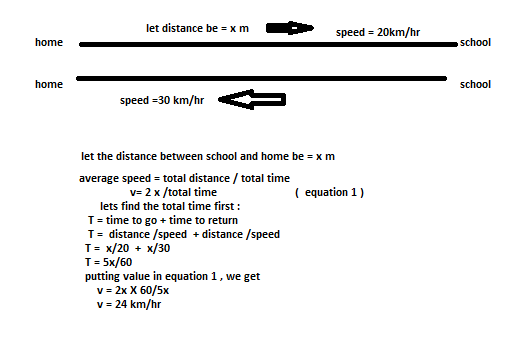Q. A ball is gently dropped from a height of 20 m. If its velocity increases uniformly at the rate of10m/sec2, with what velocity will it strike the ground? After what time will it strike the ground?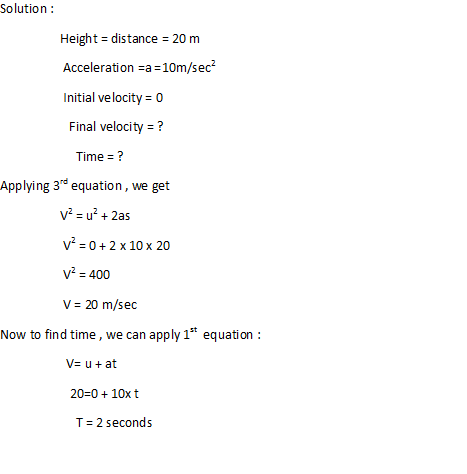Q. An object has moved through a distance. Can it have zero displacement? If so support your answer with example?
Ans.: Yes, displacement can be zero even if the distance is not zero.
Example: A child going to school in the morning and returning home after school covers distance but displacement is zero.

Q. A farmer is moving along a boundary of square field of side 10 m in 40 seconds. What will be the magnitude of displacement of the farmer at the need of 2 minutes and 20 seconds?
Solution: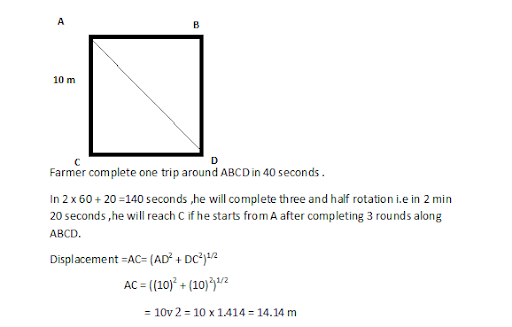Q. Which of the following is true for displacement?

1. �It can�t be zero
2. Its magnitude is greater than the distance travelled by object?

Ans.: Both are false. Firstly, it can’t be zero, positive or negative.
Secondly its magnitude is less than or equal to distance covered.

Q. Distinguish between speed and velocity?
Ans.:

 Speed Velocity It is distance travelled with respect to time in any direction. It is distance travelled with respect to time in given direction. Scalar quantity Vector quantity Complete quantity Complete quantity

Q. Under what condition is the magnitude of the average velocity of an object equal to average speed?
Ans.: The magnitude of the average velocity of an object equal to average speed when motion is along a straight line.

Q. What does the odometer measure?
Ans.: Odometer measures the distance covered .

Q. What does the path of an object look when it is in uniform motion?
Ans.: the body will displaced equally in equal intervals of time .

Q. During an experiment, a signal from a spaceship reached the ground station in 5 minute. what was the distance of spaceship from the ground station? The signal travels at a speed of light i.e. 3 x 10 8 m/sec?Q. When will you say that body is in uniform acceleration and non uniform acceleration?
Ans.: Uniform acceleration - if change in velocity is equal in equal intervals of time. Non uniform acceleration - if change in velocity is not equal in equal intervals of time.

Q. A bus decreases its speed from 80km/hr to 60km/hr In 5 sec. Find acceleration?
Ans.: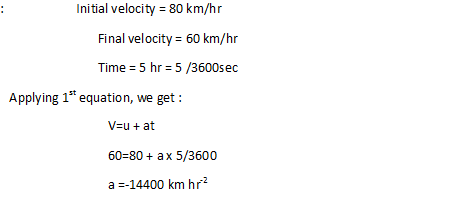Q. A train starting from railway station and moving with uniform acceleration attains the speed of 40km/hr in 10 minutes find acceleration?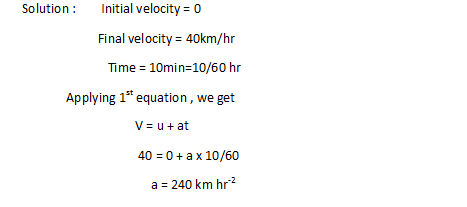Q. What is the nature of distance time graph for uniform and non uniform motion?
Ans.: For uniform motion: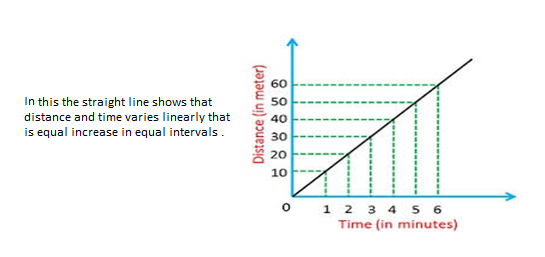For non uniform motion: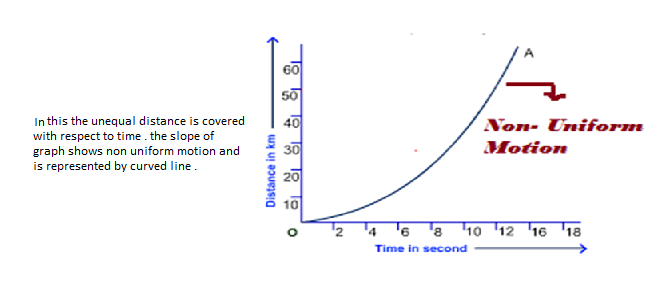Q. What can you say about the motion of object whose distance time graph is a straight line parallel to time axis?

Ans.:In this body is not changing its position with respect to time that shows body is stationary. Like if we take the markings on the axis, we see that in any time the distance covered is exactly the same.

Q. What can you say about the motion of object whose velocity time graph is a straight line parallel to time axis?
Ans.: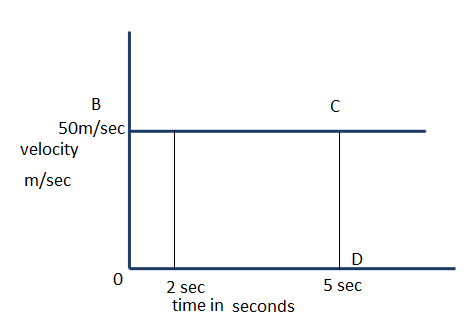In this, the body is not changing its velocity with respect to time that shows body is moving with the same velocity. Like if we take the markings on the axis, we see that in any time the velocity is exactly the same.
So, the information that we get, is:

• The body is moving with constant velocity
• Acceleration = change in velocity/time

=0/5-2=0m/sec2

• The displacement =area under v-t graph

S= area of rectangle
= OBCD
= L x B= 50 x 5 = 250m

• This shows body is starting from rest & then its velocity increases at a uniform rate.

Q. What is the quantity which is measured by the area occupied below the velocity time graph?
Ans.: The area occupied below the velocity time graph gives distance covered by the body .

Q. A bus starting from rest moves with uniform acceleration of 0.1m/sec2 for 2 minutes. Find the speed acquired and distance travelled?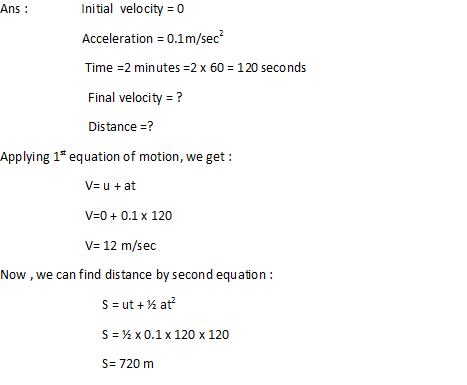Q. A train is travelling at a speed of 90km/hr. Brakes is applied so as to produce a uniform acceleration of -0.5m/sec2 . Find how far the train will go before it is brought to rest?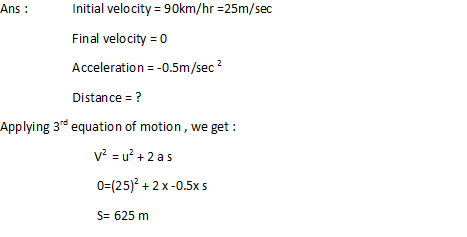Q. A trolley while going down an inclined plane has an acceleration of 2cm/sec 2.. What will be its velocity 3seconds after the start?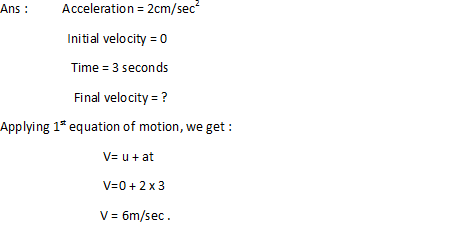Q. A racing car has uniform acceleration of 4m/sec2 . What distance will it cover in 10seconds after the start?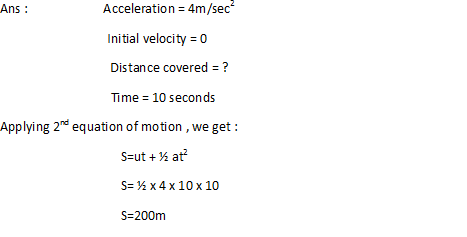Q. A stone is thrown vertically upward with a velocity of 5m/sec. If the acceleration of stone during its motion is 10m/sec 2 in the downward direction, What will be the height attained by the stone and how much time will it take to reach there?Q. An athlete covers one round of circular track of diameter 200m in 40 seconds. What will be the distance covered and displacement at the end of 2 minutes 30 seconds?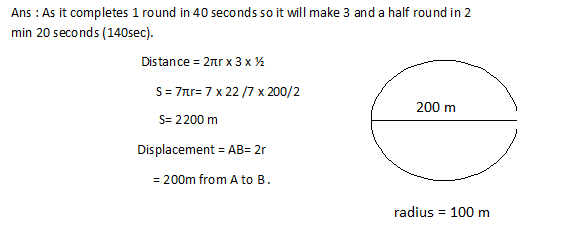Q. A motor boat starting from rest on a lake accelerates in a straight line at constant rate of 3m/sec 2 for 8 seconds. How far does the boat travel during this time?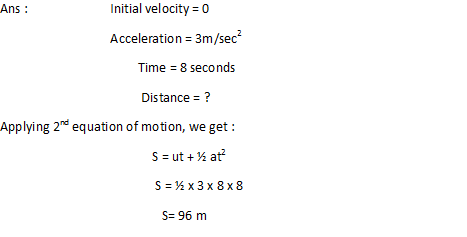Q. An artificial satellite is moving in a circular orbit of radius 42,250 km. Calculate its speed if it takes 24 hours to revolve around the earth?
Ans.: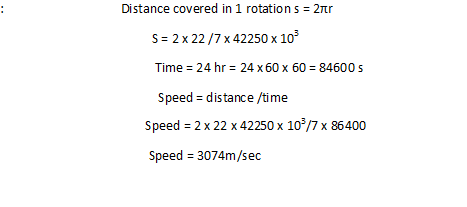Q. State which of the following situations are possible and give an example of each:

• An object with constant acceleration but zero velocity
• An object moving in a certain direction with acceleration in perpendicular direction?

Ans.: (a) when a body is projected upwards, its velocity at highest point reached is zero although it has uniform acceleration.
(b) When an object is moving in a circular path, its direction of motion is towards tangent to the circle although the centripetal acceleration is acting perpendicular to the direction of motion that is towards the circle.

Q. The speed time graph shown for the car is shown in figure:
How far does the car travel in first 4 seconds?
Which part of the graph represents uniform motion?
Ans.: The Area OABO gives the distance in first 4 seconds
Part CD gives uniform motion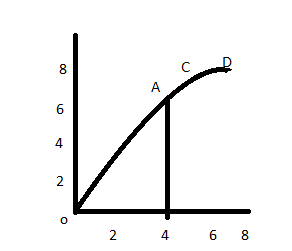Q. A driver of a car travelling at 52km/hr applies brakes and accelerates uniformly in the opposite direction the car stops in 5 seconds. Another driver going at 34km/hr in another car applies brakes slowly and stops in 10 seconds. Plot the speed versus time graph for both time graphs for two cars. Which of the two travels farther after the brakes are applied?